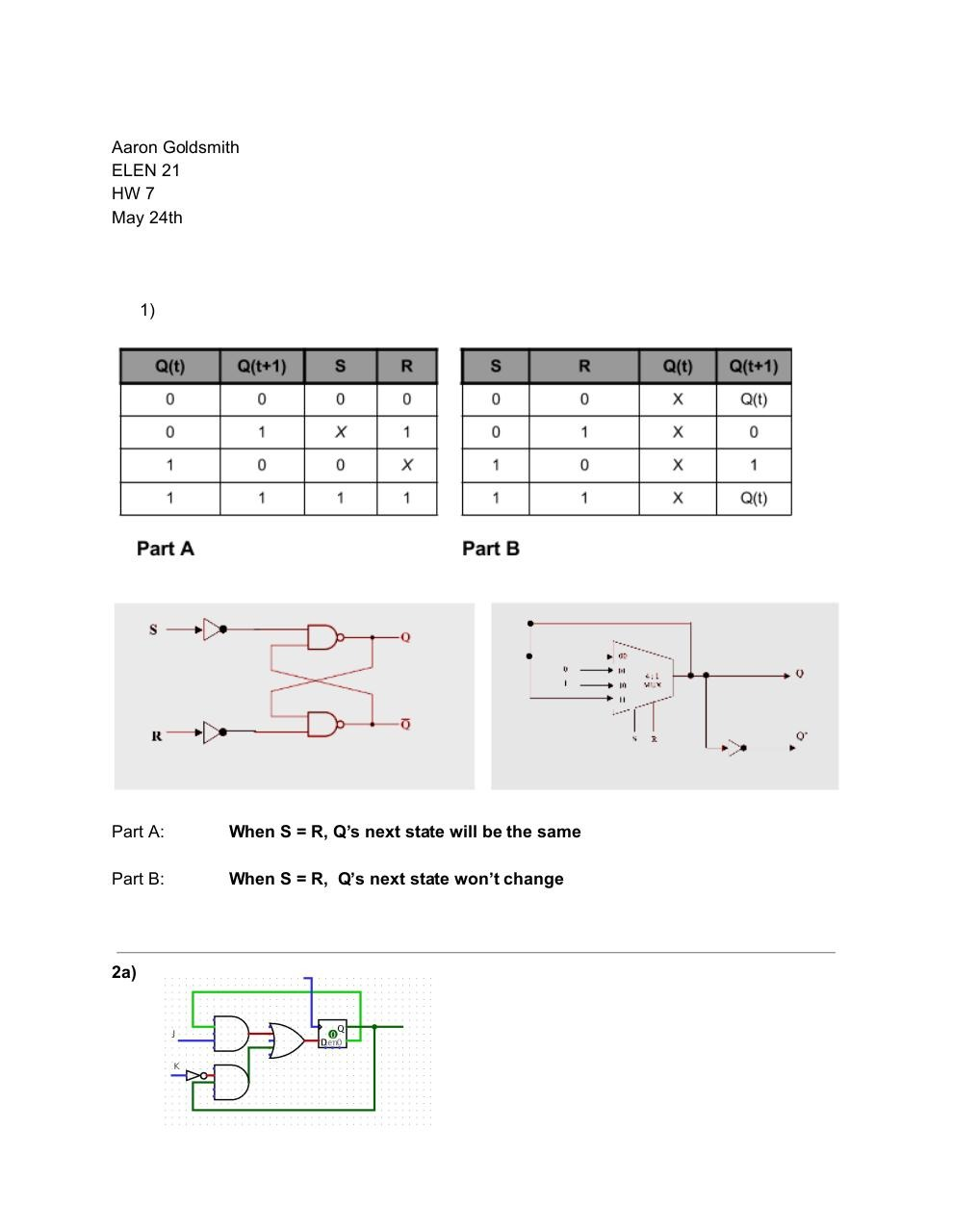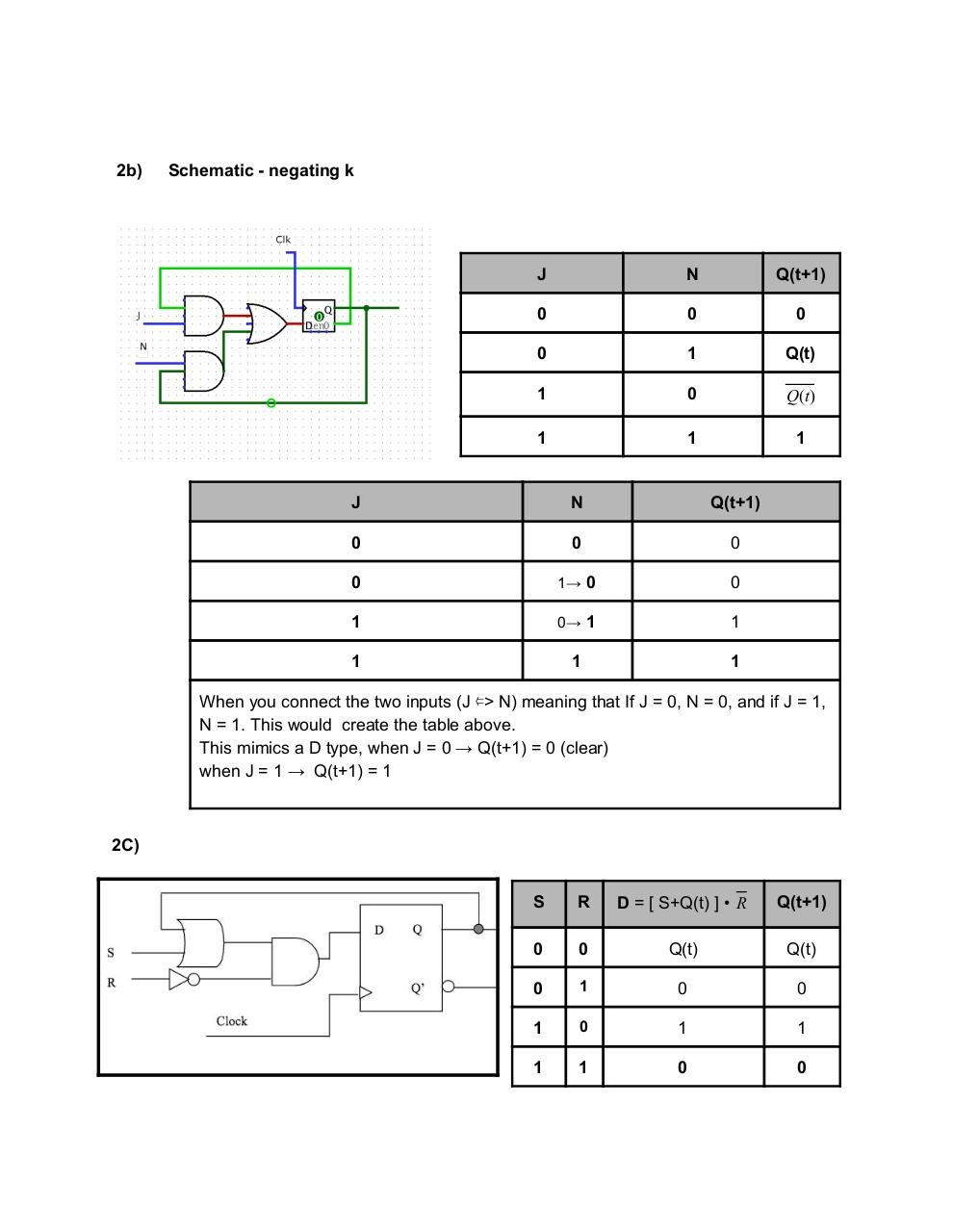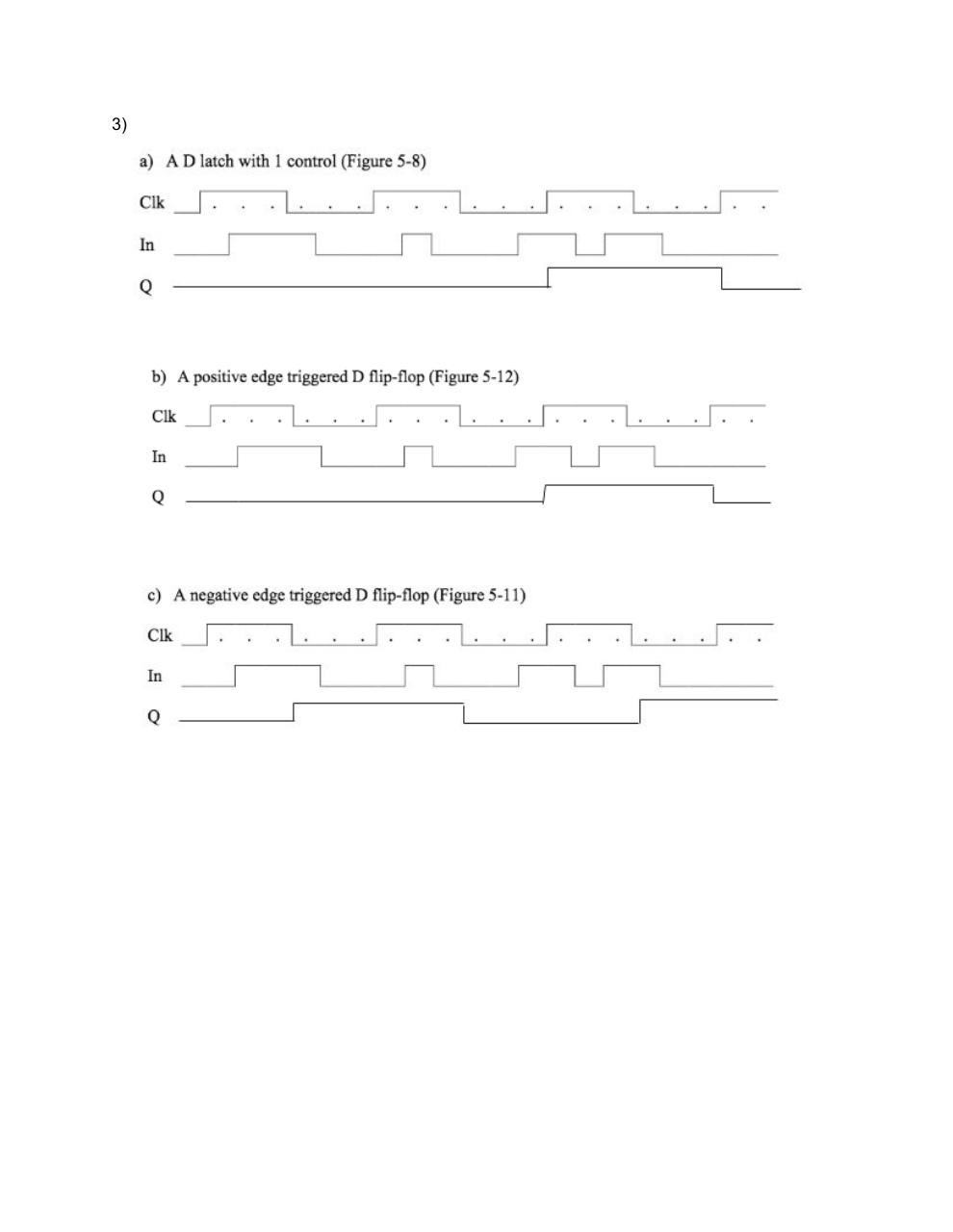# AaronGoldsmith .pdf

### File information

Original filename: AaronGoldsmith.pdf

This PDF 1.5 document has been generated by / Skia/PDF m52, and has been sent on pdf-archive.com on 25/05/2016 at 05:32, from IP address 174.62.x.x. The current document download page has been viewed 257 times.
File size: 273 KB (3 pages).
Privacy: public file

AaronGoldsmith.pdf (PDF, 273 KB)

### Document preview

Aaron Goldsmith
ELEN 21
HW 7
May 24th

1)

Part A:

Part B:

​
When S = R, Q’s next state will be the same
​
When S = R, ​
​
Q’s next state won’t change

2a)

2b)

Schematic ­ negating k

Q(t+1)

Q(t)

Q(t)

Q(t+1)

1→​
0

0→​
1

When you connect the two inputs (J ⇐
&gt; N) meaning that If J = 0, N = 0, and if J = 1,
N = 1. This would  create the table above.
This mimics a D type, when J = 0 → Q(t+1) = 0 (clear)
when J = 1 →  Q(t+1) = 1

2C)

D ​
= [ S+Q(t) ] •  R

Q(t+1)

Q(t)

Q(t)

3)#### HTML Code

Copy the following HTML code to share your document on a Website or Blog

#### QR Code### Related keywords